Important Questions on Circles

Chapter 9 Class 9 Circles
Serial order wise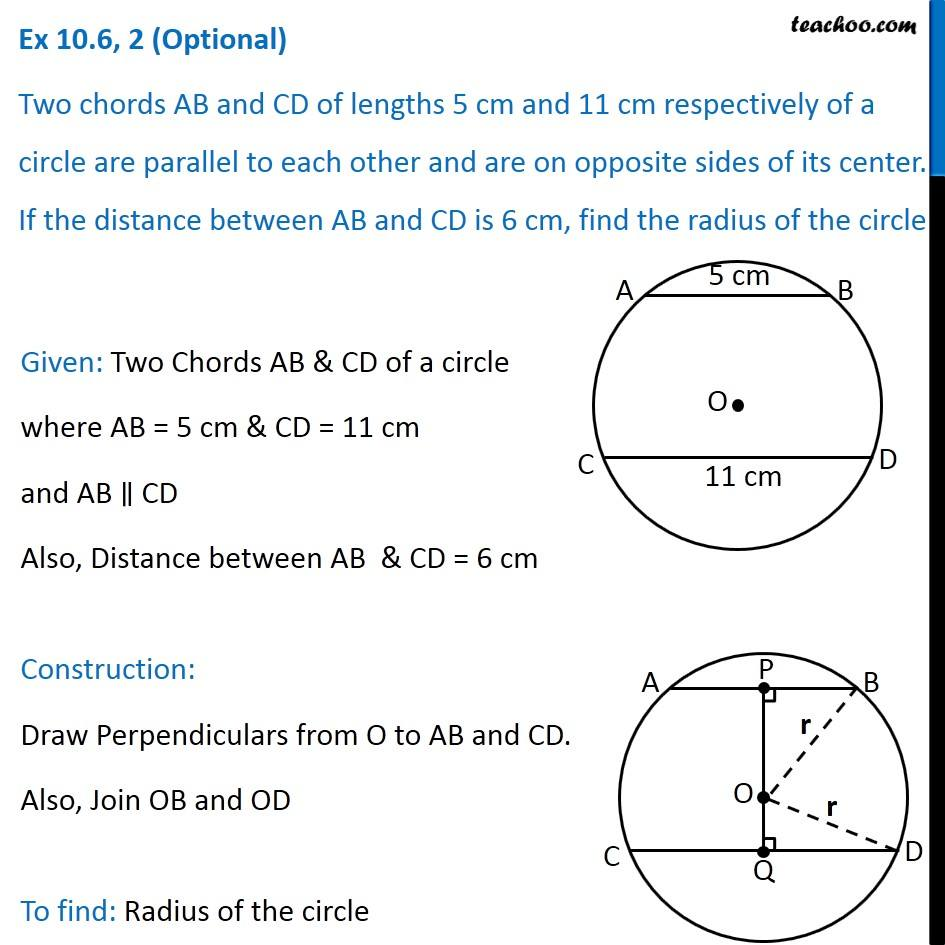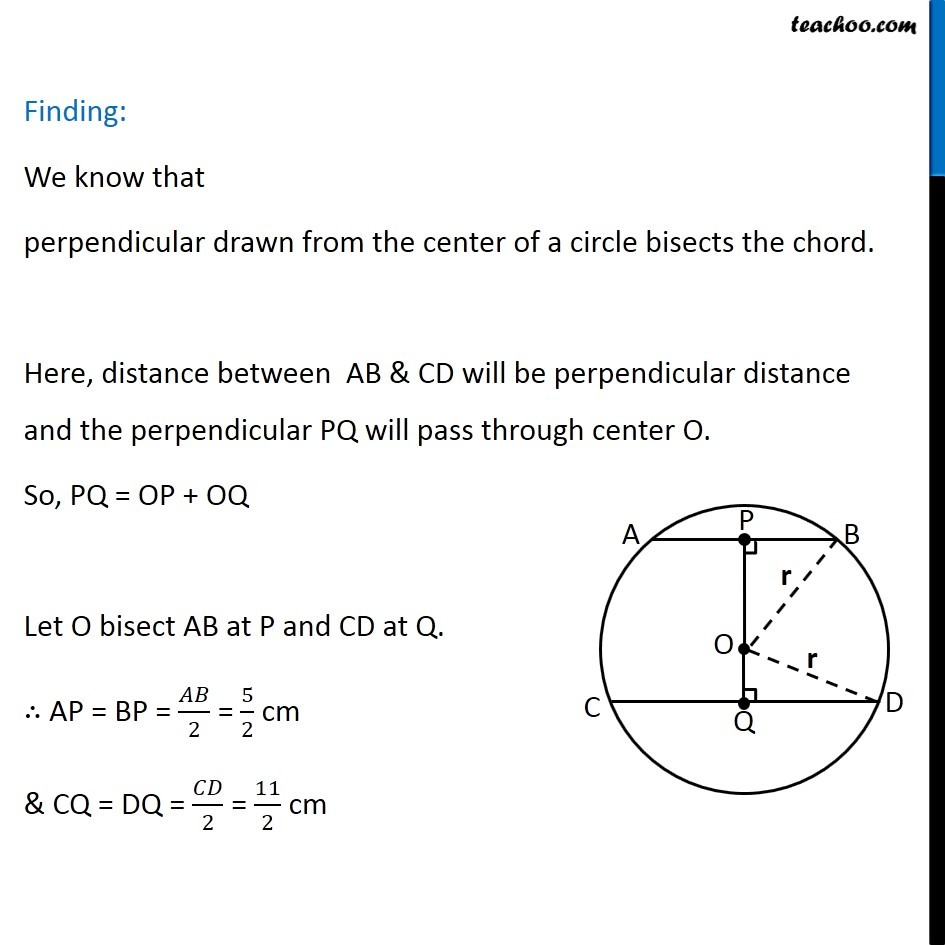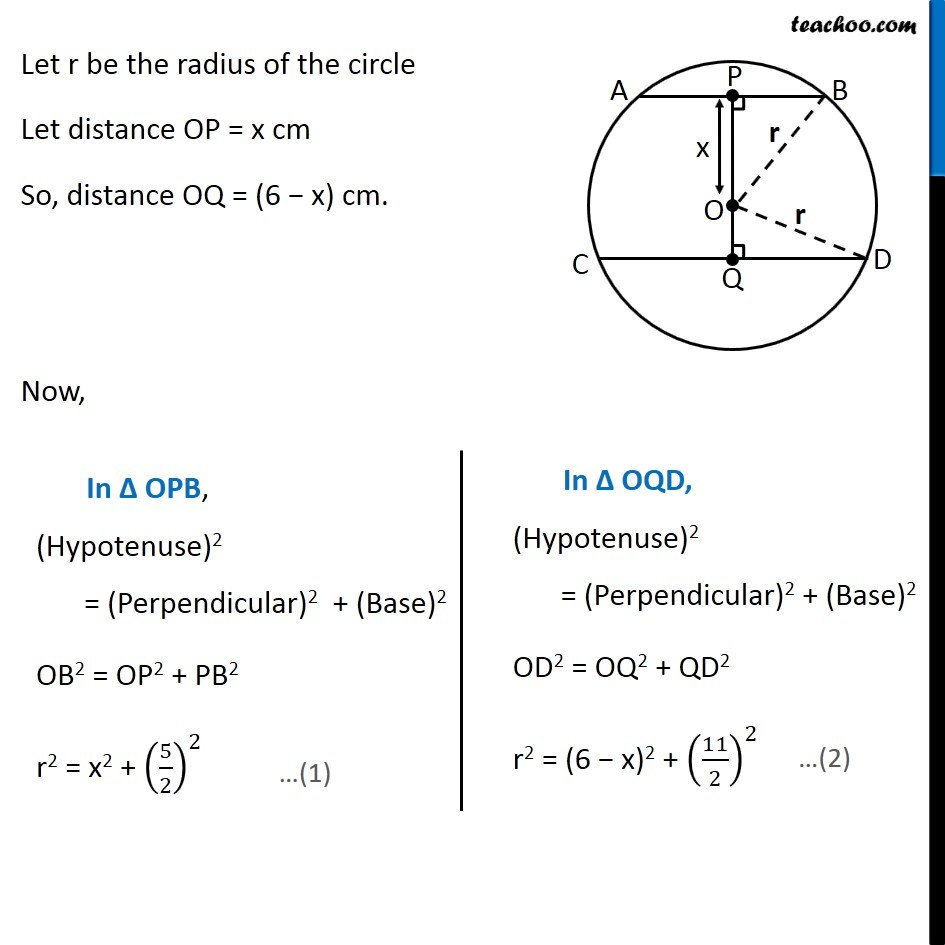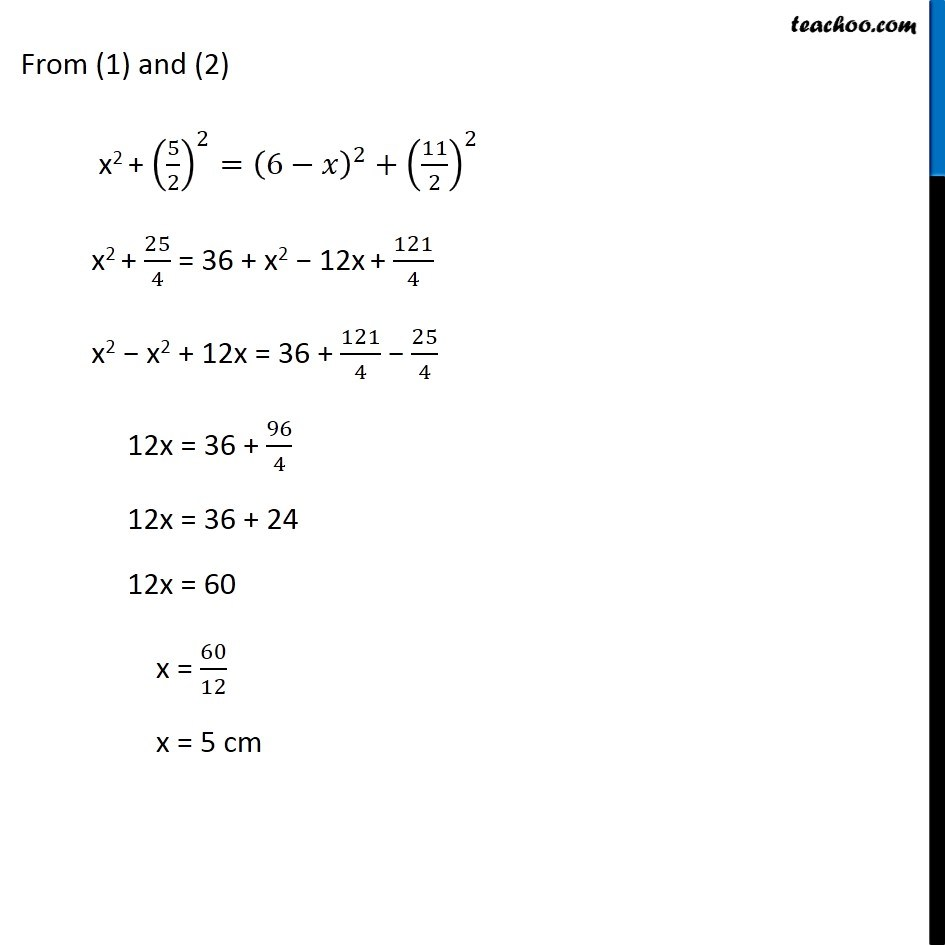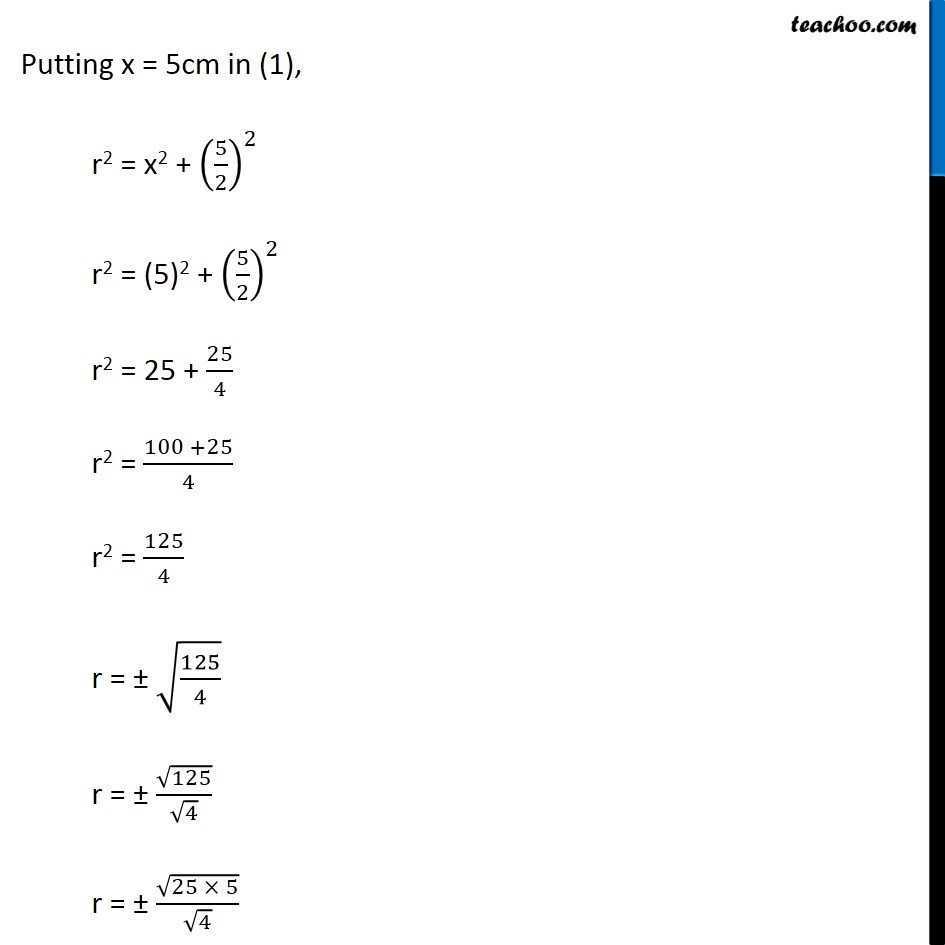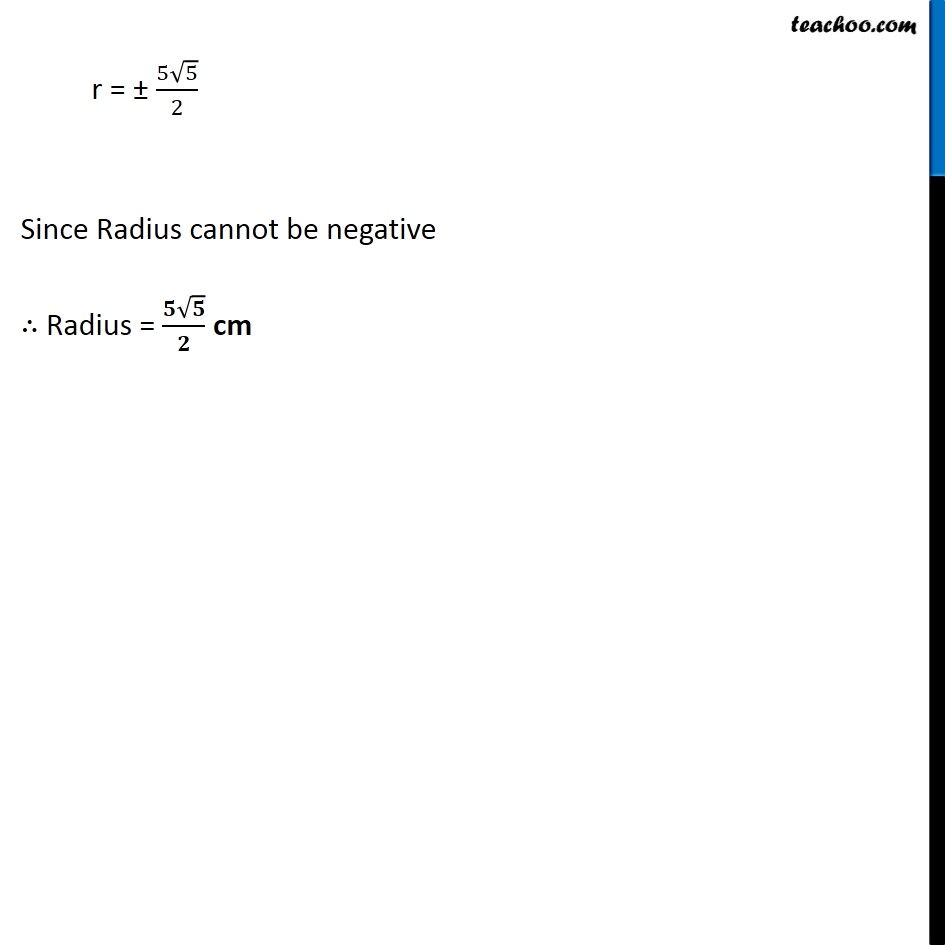Learn in your speed, with individual attention - Teachoo Maths 1-on-1 Class

### Transcript

Question 2 Two chords AB and CD of lengths 5 cm and 11 cm respectively of a circle are parallel to each other and are on opposite sides of its center. If the distance between AB and CD is 6 cm, find the radius of the circle Given: Two Chords AB & CD of a circle where AB = 5 cm & CD = 11 cm and AB ∥ CD Also, Distance between AB & CD = 6 cm Construction: Draw Perpendiculars from O to AB and CD. Also, Join OB and OD To find: Radius of the circle Finding: We know that perpendicular drawn from the center of a circle bisects the chord. Here, distance between AB & CD will be perpendicular distance and the perpendicular PQ will pass through center O. So, PQ = OP + OQ Let O bisect AB at P and CD at Q. ∴ AP = BP = 𝐴𝐵/2 = 5/2 cm & CQ = DQ = 𝐶𝐷/2 = 11/2 cm Let r be the radius of the circle Let distance OP = x cm So, distance OQ = (6 − x) cm. Now, In ∆ OPB, (Hypotenuse)2 = (Perpendicular)2 + (Base)2 OB2 = OP2 + PB2 r2 = x2 + (5/2)^2 …(1) In ∆ OQD, (Hypotenuse)2 = (Perpendicular)2 + (Base)2 OD2 = OQ2 + QD2 r2 = (6 − x)2 + (11/2)^2 …(2) From (1) and (2) x2 + (5/2)^2=(6−𝑥)^2+(11/2)^2 x2 + 25/4 = 36 + x2 − 12x + 121/4 x2 − x2 + 12x = 36 + 121/4 − 25/4 12x = 36 + 96/4 12x = 36 + 24 12x = 60 x = 60/12 x = 5 cm Putting x = 5cm in (1), r2 = x2 + (5/2)^2 r2 = (5)2 + (5/2)^2 r2 = 25 + 25/4 r2 = (100 +25)/4 r2 = 125/4 r = ± √(125/4) r = ± √125/√4 r = ± √(25 × 5)/√4 r = ± (5√5)/2 Since Radius cannot be negative ∴ Radius = (𝟓√𝟓)/𝟐 cm